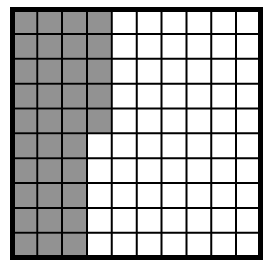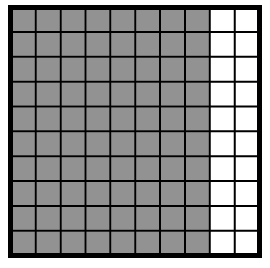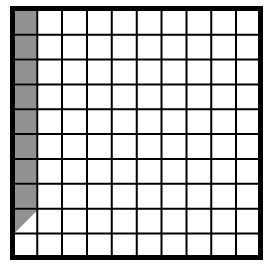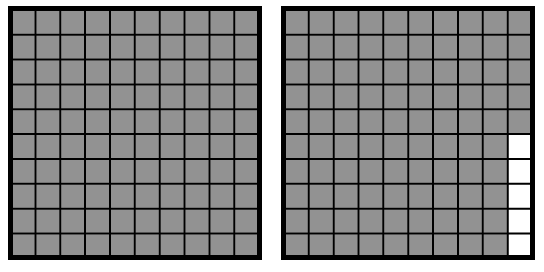Home > CC1MN > Chapter cc13 > Lesson cc13.1.4 > Problem3-64

3-64.

For each of the representations below, write the portion in each of the forms listed. Remember that a hundred block now represents $100\%$. Homework Help ✎

• Percent

• Decimal

• Fraction

• Description in words

1.3 full columns out of 10 are shaded, plus half of another column. How can you write this in the forms listed above?

Percent: $35\%$

$\text{Fraction: }\frac{35}{100} \text{ or }\frac{7}{20}$

Decimal: $0.35$

Words: Thirty-five hundredths or three tenths and five hundredths or thirty-five out of one hundred

1.This problem is similar to part (a).

1.• To represent five and one-half hundredths,

$\text{you would write 5.5%, }\ \frac{55}{1000},\text{ or } 0.055.$

1.• Since there are two $100\%$ blocks in this problem, the answers will be greater than $100\%$.
In percent form, this is$195\%$. Can you write this as a decimal, fraction, and in words?